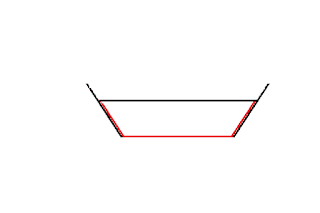# Wetted Perimeter

Wetted perimeter refers to the perimeter of the surface of the pipe in contact with the medium.  If the pipe is circular, the wetted perimeter will be 2pir

If the pipe has a square cross-section, the wetted perimeter will be 4a.Wetted Area of a Trapezoidal channel in Red

The wetted perimeter for regular geometric shaped conduits can be calculated using standard formula.

For irregular conduits such as rivers and channels, the wetted perimeter has to be calculated manually.  To measure the wetted perimeter of rivers, a long chain is suspended across the river from either bank.  Using a boat, the depth of the river is measured every 50 cms and the values are noted.  This data can be drawn and the wetted area can be calculated.